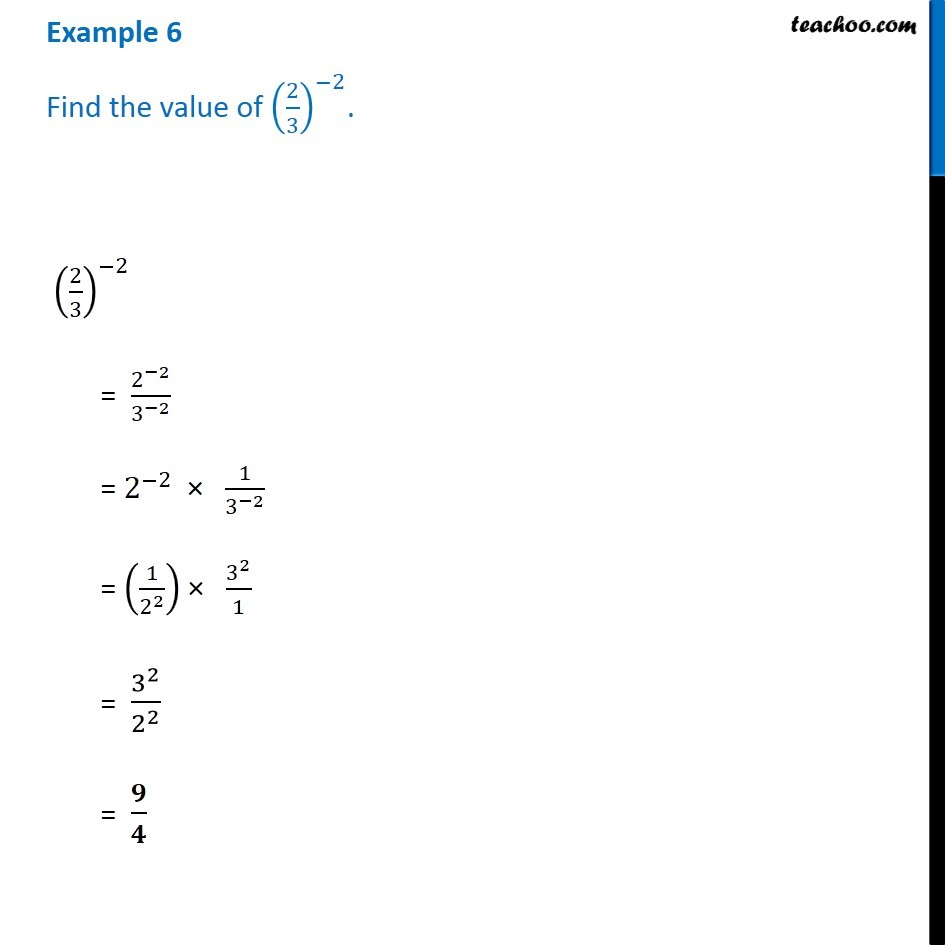1. Chapter 12 Class 8 Exponents and Powers
2. Concept wise
3. Exponents

Transcript

Example 6 - Chapter 12 Class 8 Exponents and Power - NCERT Solution (2/3)^(−2) = 2^(−2)/3^(−2) = 2^(−2) × 1/3^(−2) = (1/2^2 ) × 3^2/1 = 3^2/2^2 = 𝟗/𝟒

Exponents